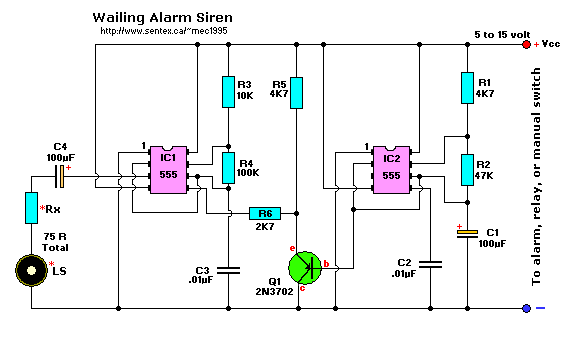```Parts List:
R1,R5 = 4.7K            C1,C4 = 100uF/25V, electrolytic
R2 = 47K             C2,C3 = 0.01uF (10nF), ceramic
R3 = 10K                Q1 = 2N3702 (NTE159, TUP, etc.)
R4 = 100K          IC1,IC2 = LM555, NE555, uA555
*Rx = See text           LS = Loudspeaker (see text)

A complete KIT plus Printed Circuit Board will be available soon.

```
Notes:
This circuit provides a warbling sound to any alarm circuit. IC2 is wired as a low frequency astable with a cycle period of about 6 seconds. The slowly varying ramp waveform at C1 is fed to PNP emitter follower Q1, and is then used to frequency modulate alarm generator IC1 via R6. IC1 has a natural center frequency of about 800Hz.
Circuit action is such that the alarm output signal starts at a low frequency, rises for 3 seconds to a high frequency, then falls over 3 seconds to a low frequency again, and so on.

* The Loudspeaker LS and the resistor marked "Rx" should be together 75 ohms. If you have a standard 8-ohm speaker then Rx is 67 ohms. The nearest value is 68 ohms. So for a 8 ohm loudspeaker Rx is 68 ohms. For a 4 ohm loudspeaker Rx is 71 ohms, for a 25 ohm loudspeaker Rx is 50 ohms, etc. The Rx value is not very critical. It is just there as some sort of volume control, experiment with it.

C2 and C3 are 0.01uF (10nF) and a simple ceramic type will do the job. I tested the circuit at 9, 12, and 15 volt. My personal choice is the 9 volt alkaline type for battery operation, or 12 volt for use with a small powersupply. The so-called 9V NiCad will not work properly. The actual voltage for this NiCad type is actually only 7.5V (6 cells x 1.2V = 7.5V)

Just in case you're wondering, output pin 3 of IC2 is NOT connected! In the prototype, I used LM555 timers.

Back to Circuits Page